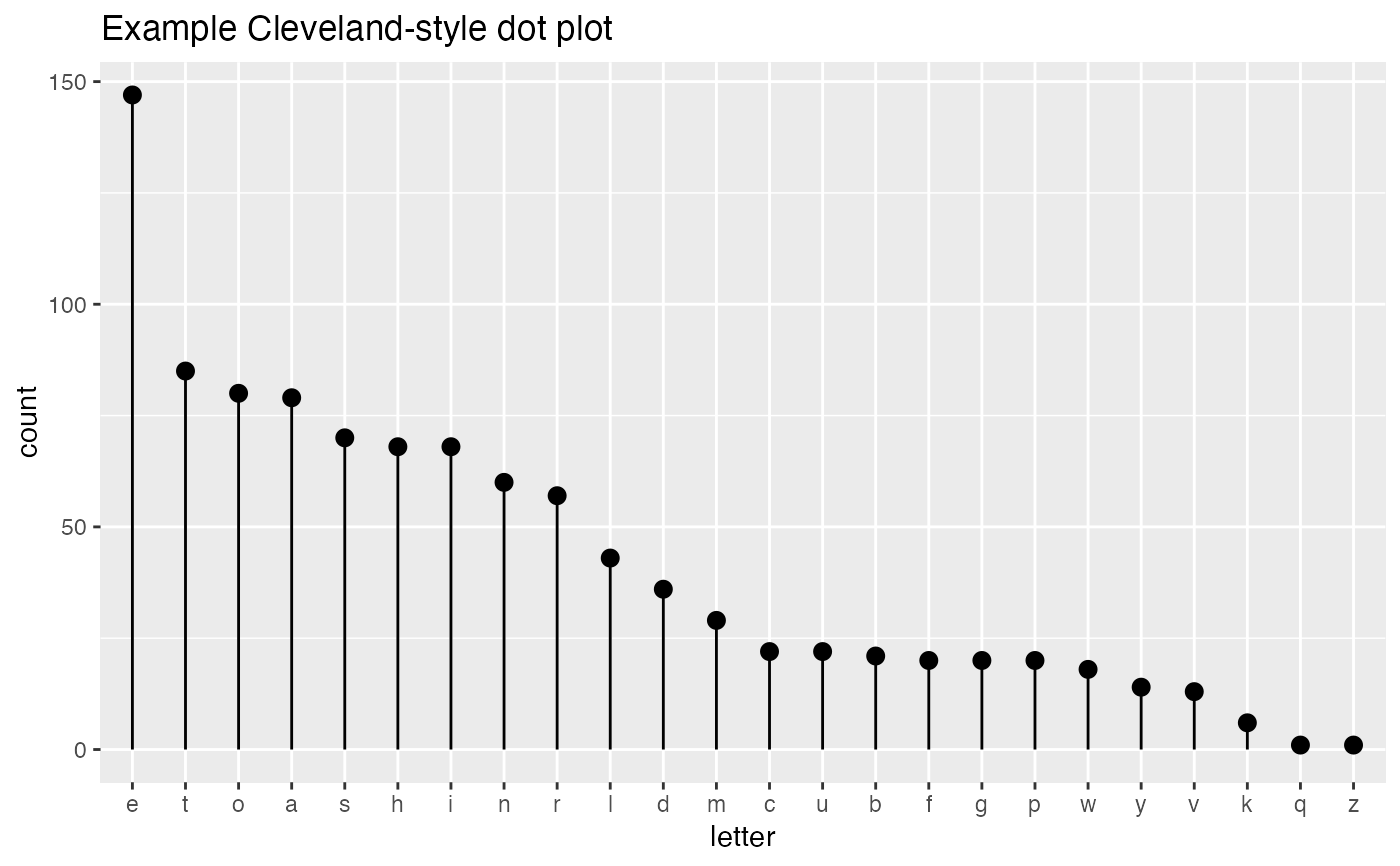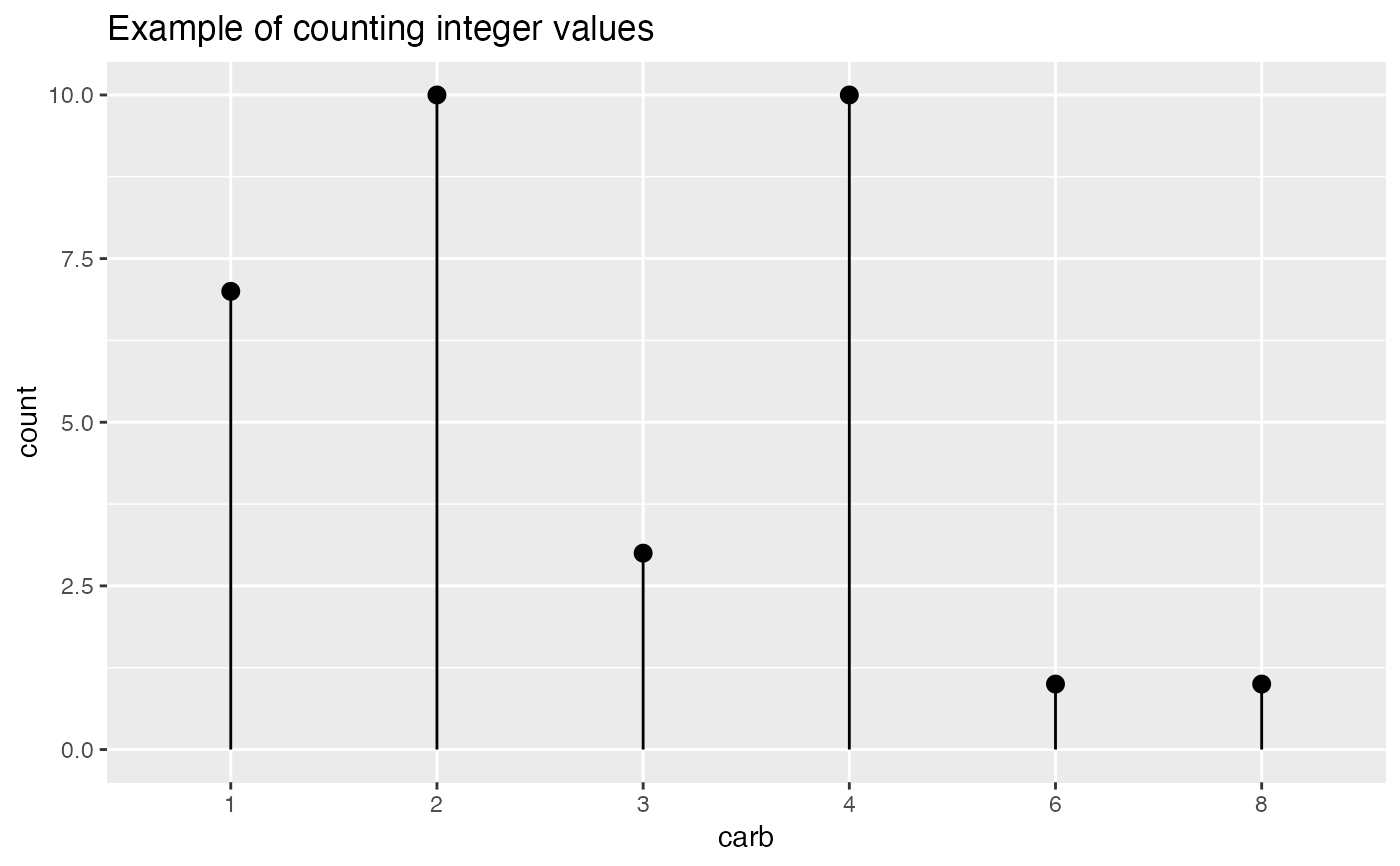Plot counts of a categorical variable.

ClevelandDotPlot(
frm,
xvar,
title,
...,
sort = -1,
limit_n = NULL,
stem = TRUE,
color = "black"
)

## Arguments

frm data frame to get values from name of the independent (input or model) column in frame title to place on plot no unnamed argument, added to force named binding of later arguments. if TRUE sort data if not NULL number of items to plot if TRUE add stems/whiskers to plot color for points and stems

## Details

Assumes that xvar is a factor or can be coerced to one (character or integral).

• sort < 0 sorts the factor levels in decreasing order (most frequent level first)

• sort > 0 sorts the factor levels in increasing order (good when used in conjunction with coord_flip())

• sort = 0 leaves the factor levels in "natural order" -- usually alphabetical

• stem = FALSE will plot only the dots, without the stem to the y=0 line.

• limit_n = NULL plots all the levels, N an integer limits to the top N most populous levels

## Examples


set.seed(34903490)
# discrete variable: letters of the alphabet
# frequencies of letters in English
# source: http://en.algoritmy.net/article/40379/Letter-frequency-English
letterFreqs = c(8.167, 1.492, 2.782, 4.253, 12.702, 2.228,
2.015, 6.094, 6.966, 0.153, 0.772, 4.025, 2.406, 6.749, 7.507, 1.929,
0.095, 5.987, 6.327, 9.056, 2.758, 0.978, 2.360, 0.150, 1.974, 0.074)
letterFreqs = letterFreqs/100
letterFrame = data.frame(letter = letters, freq=letterFreqs)
# now let's generate letters according to their letter frequencies
N = 1000
randomDraws = data.frame(draw=1:N,
letter=sample(letterFrame$letter, size=N, replace=TRUE, prob=letterFrame$freq))
WVPlots::ClevelandDotPlot(randomDraws, "letter",
title = "Example Cleveland-style dot plot")# Note the use of sort = 0, and that the graph correctly includes counts
# with no occurrences (5, and 7)
WVPlots::ClevelandDotPlot(mtcars, "carb", sort = 0, "Example of counting integer values")